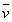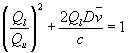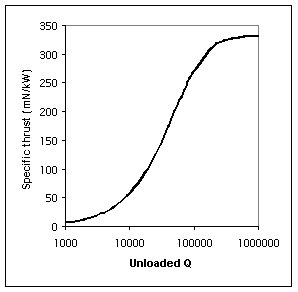SPR Ltd.

 Home Background Benefits Theory Development Applications Future FAQ

Applications

Applications of first generation engines

Any analysis of missions incorporating microwave engines will need to take into account the second important equation. This equation results from consideration of the principle of the conservation of energy and introduces the concept of the loaded Q of the engine.

The Q of any resonant circuit can be defined as the stored energy divided by the energy loss per cycle. Thus as soon as kinetic energy is extracted from the engine, the stored energy, and hence the Q, falls.

We have defined the maximum Q of the engine, under static thrust conditions as Qu, the unloaded Q. Under acceleration, the Q of the engine at an average velocity ofis defined as Ql, the loaded Q.

The relationship between loaded Q and unloaded Q is given by the equation:Equation 2.

Fig 4 gives the solution to equation 1 for a velocity of 3 km/s, where Qu is replaced with Ql, obtained from equation 2. The specific thrust for a theoretical design factor of 0.945 increases, with increasing unloaded Q, up to a maximum of 333mN/KW.Fig 4. Specific Thrust at 3 km/s.

Conventional microwave technology limits the maximum Q of resonators to around 50,000, giving a specific thrust of 200 mN/kW at 3 km/s. This technology will be utilised in first generation engines where typical applications will be the orbital transfer and orbit maintenance of communication satellites and the primary propulsion for science missions.